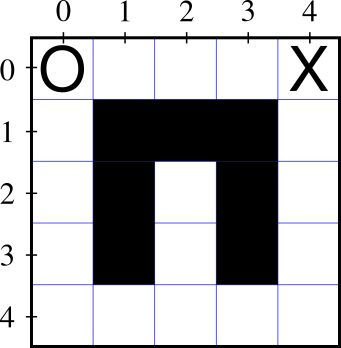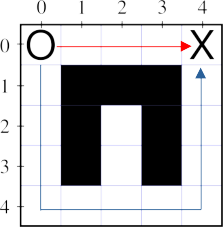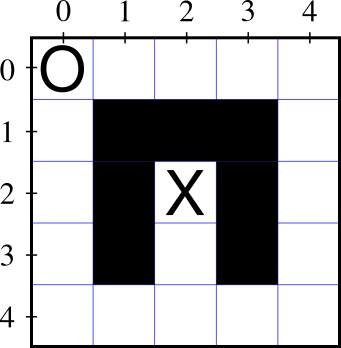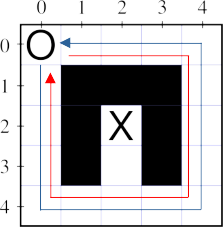CSE-250 Fall 2022 - Section B - Average Runtime, Stacks, Queues### Average Runtime, Stacks, Queues

Oct 3, 2022

#### Stacks

A stack of objects on top of one another.

Push
Put a new object on top of the stack
Pop
Remove the object on top of the stack
Top
Peek at what's on top of the stack

#### Queue

Outside of the US, "queueing" is lining up.

Enqueue
Put a new object at the end of the queue
Dequeue
Remove the next object in the queue
Peek at the next object in the queue

Thought question: How could you use an array to build a queue?

#### ArrayBuffer Attempt 1

Enqueue
Append
Dequeue
Remove(0)

What's the complexity?

#### ArrayBuffer Attempt 2

Enqueue
Insert(0)
Dequeue
Remove(last)

What's the complexity?

Can we avoid having to move all of the
elements forward or backward a spot?

_
_
_
_

#### ArrayDequeue (Resizable Ring Buffer)

Active Array = [start, end)

Enqueue
Resize buffer if needed
Advance end pointer (wrap around to front)
Dequeue
Remove element at buffer[start]
Advance start pointer (wrap around to front)

What's the complexity?

#### Applications of Stacks and Queues

• Stack: Checking for Balanced Parenthesis/Braces
• Queue: Scheduling Packets for Delivery
• Both: Searching Mazes

#### Balanced Parenthesis/Braces

What does it mean for parenthesis/braces to be balanced?

• Every opening symbol is matched by a closing symbol
• No nesting overlaps (e.g., {(}) is not ok).
 {()({})} {()) ()) ✅ ❌ ❌

#### Balanced Parenthesis/Braces

Idea: Count the number of unmatched open parens, braces.

Increment counter on (, decrement on )

Problem: allows {(})

#### Balanced Parenthesis/Braces

Idea: Track nesting on a stack!

On ( or {, push the symbol on the stack.

On ) or }, pop the stack and check the popped symbol.

#### Network Packets

Router: 1gb/s internal network, 100mb/s external
• 1gb/s sent to router, but only 100mb/s can leave.
• How do packets get delivered?
Queues
• Enqueue data packets in the order they are received.
• When outgoing bandwidth available, dequeue and send.
Avoiding Queueing Delays
• Limit size of queue; Packets that don't fit are dropped

TCP: blocked packets are retried UDP: application deals with dropped packets.

#### Mazes

• O is the start, X is the objective
• There may be multiple paths
• Generally, we want the shortest
• Approach 1: Take the first available route in one direction
• Right, Down, Left, or Up
• Down, Right, Up, Left#### Mazes

• O is the start, X is the objective
• There may be multiple paths
• Generally, we want the shortest
• Approach 1: Take the first available route in one direction
• Right, Down, Left, or Up
• Down, Right, Up, Left#### MazesHow do you know which one is best?

Other problems with this algorithm?

#### Mazes#### MazesPriority order doesn't guarantee exploring the entire maze

#### Formalizing Maze-Solving

Inputs:

• The map: An $n\times m$ grid of filled/empty squares.
• The O is at position $start$
• The X is at position $dest$

Goal:

• Compute $steps(\texttt{start}, \texttt{dest})$, the minimum steps from start to end.
• How do we define $steps$?

#### Formalizing Maze-Solving

$$steps(\texttt{pos},\texttt{dest}) = \begin{cases} 0 & \textbf{if } \texttt{pos} = \texttt{dest}\\ \end{cases}$$

#### Formalizing Maze-Solving

$$steps(\texttt{pos},\texttt{dest}) = \begin{cases} 0 & \textbf{if } \texttt{pos} = \texttt{dest}\\ \infty & \textbf{if } is\_filled(\texttt{pos})\\ \end{cases}$$

#### Formalizing Maze-Solving

$$steps(\texttt{pos},\texttt{dest}) = \begin{cases} 0 & \textbf{if } \texttt{pos} = \texttt{dest}\\ \infty & \textbf{if } is\_filled(\texttt{pos})\\ 1 + min\_adjacent(\texttt{pos}, \texttt{dest}) & \textbf{otherwise} \end{cases}$$

where...

$min\_adjacent(\texttt{pos}, \texttt{dest}) =$ $$\min\begin{cases} steps(moveRight(\texttt{pos}), \texttt{dest})\\ steps(moveDown(\texttt{pos}), \texttt{dest})\\ steps(moveLeft(\texttt{pos}), \texttt{dest})\\ steps(moveUp(\texttt{pos}), \texttt{dest})\\ \end{cases}$$

#### $steps(\texttt{pos}, \texttt{dest})$

• if pos == dest then return 0
• elif is_filled(pos) then return ∞
• else return 1 + min of
• $steps(moveRight(\texttt{pos}, \texttt{dest}))$
• $steps(moveDown(\texttt{pos}, \texttt{dest}))$
• $steps(moveLeft(\texttt{pos}, \texttt{dest}))$
• $steps(moveUp(\texttt{pos}, \texttt{dest}))$Problem: Infinite loop!

Insight: A path with a loop in it can't
be shorter than one without the loop.

Mark nodes as visited

#### $steps(\texttt{pos}, \texttt{dest})$

• if pos == dest then return 0
• elif pos marked visited then return ∞
• elif is_filled(pos) then return ∞
• else
• Mark pos as visited
• return 1 + min of all 4 stepsProblem: The first time you visit a node,
it may be via a longer path!

Unmark nodes as you leave them

#### $steps(\texttt{pos}, \texttt{dest})$

• if pos == dest then return 0
• elif pos marked visited then return ∞
• elif is_filled(pos) then return ∞
• else
• Mark pos as visited
• stepCount = 1 + min of all 4 steps
• Unmark pos as visited
• return stepCount

#### Maze-Solving

Inputs:

• The map: An $n\times m$ grid of filled/empty squares.
• The O is at position $start$
• The X is at position $dest$

Goal:

• Compute $steps(\texttt{start}, \texttt{dest})$, the minimum steps from start to end.
• What path did we take?

Idea:

#### $steps(\texttt{pos}, \texttt{dest}, \texttt{visited})$

• if pos == dest then return visited.copy()
• elif pos visited return no_path
• elif is_filled(pos) then return no_path
• else
• visited.append(pos)
• bestPath = min of all 4 one-step paths
• visited.removeLast()
• return bestPath

#### $steps(\texttt{pos}, \texttt{dest}, \texttt{visited})$

• if pos == dest then return visited.copy()
• elif posvisited return no_path
• elif is_filled(pos) then return no_path
• else
• visited.push(pos)
• bestPath = min of all 4 one-step paths
• visited.pop()
• return bestPath

Thought question: Can you solve a maze